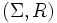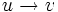# Rewriting system

Jump to: navigation, search

## Definition

A rewriting system$(\Sigma,R)$ is the following data:

• A set$\Sigma$ called the set of generators or the alphabet (its elements are the letters). The set of words (sequences of finite length) is termed$\Sigma^*$.
• A subset$R$ of$\Sigma^* \times \Sigma^*$. Each element of this subset is termed a rewrite

Every rewriting system gives a presentation of a monoid as follows: the generating set is simply$\Sigma$, and the set of relations is simply that obtained by declaring that$u = v$ for any pair$(u,v) \in R$.

Also refer rewriting system for a group.

## Terminology

### Reduction

Given a rewriting system$(\Sigma,R)$, a reduction for this rewriting system, is a pair$(u,v) \in \Sigma^* \times \Sigma^*$ such that there exist$a,l,b,r \in \Sigma^*$ such that$(l,r) \in R$,$u = alb$ and$v = arb$.

A reduction is said to be the identity reduction if$l=r$.

Note that just saying$u = v$ does not mean that the reduction is the identity reduction.

The pair$(l,r)$ is termed the rewrite rule associated with the reduction.

A reduction$(u,v)$ is typically denoted as$u \to v$. We often write reductions in sequence. For instance, if$(u,v)$ is a reduction and$(v,w)$ is a reduction, then we write:$u \to v \to w$

We may even branch out multiple reductions. For instance, if$(u,v_1)$ and$(u,v_2)$ are reductions, then we can depict this by a diagram with an arrow from$u$ to$v_1$ and an arrow from$u$ to$v_2$.

### Irreducible word

An irreducible word is a word such that the only reduction from it (if any) is the identity reduction.

## Facts

### Properties of rewriting systems

For a full list of properties of rewriting systems, refer:

## =Constructs

### Free product of monoids

Given a free product of monoids, a rewriting system for the free product can be obtained by simply taking the union of the corresponding generating sets and rewrites, after first disjointifying the alphabets.

### Direct product of monoids

Given a direct product of monoids, a rewriting system for the direct product can be obtained as follows:

• First take the rewriting system for the product
• Now introduce a total ordering among the direct factors
• Now introduce rewrites of the form$ab \to ba$ whenever$a$ and$b$ are letters in the direct factors such that the factor in which$a$ lies, come after the factor in which$b$ lies.

In other words, these relations help us bubble all the letters of the first factor to the left, the letters of the second factor after that, the letters of the third factor after that, and so on.

### Quotient

A rewriting system for the quotient of a monoid by some congruence can be described if we have a collection of relations generating the congruence. In that case, we simply direct each relation in any way we want and get a collection of rewrites.